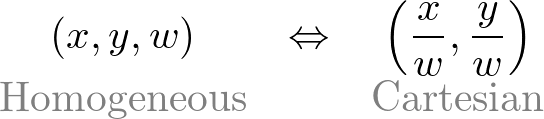Homogeneous Coordinates - Tech It Yourself

## Sunday, 10 November 2019

### Problem: Two parallel lines can intersect.Railroad gets narrower and meets at horizon.
In Euclidean space (geometry), two parallel lines on the same plane cannot intersect, or cannot meet each other forever. It is a common sense that everyone is familiar with.

However, it is not true any more in projective space, for example, the train railroad on the side picture becomes narrower while it moves far away from eyes. Finally, the two parallel rails meet at the horizon, which is a point at infinity.

Euclidean space (or Cartesian space) describe our 2D/3D geometry so well, but they are not sufficient to handle the projective space (Actually, Euclidean geometry is a subset of projective geometry). The Cartesian coordinates of a 2D point can be expressed as (x, y).

What if this point goes far away to infinity? The point at infinity would be (∞,∞), and it becomes meaningless in Euclidean space. The parallel lines should meet at infinity in projective space, but cannot do in Euclidean space. Mathematicians have discoverd a way to solve this issue.

### Solution: Homogeneous Coordinates

Homogeneous coordinates, introduced by August Ferdinand Möbius, make calculations of graphics and geometry possible in projective space. Homogeneous coordinates are a way of representing N-dimensional coordinates with N+1 numbers.

To make 2D Homogeneous coordinates, we simply add an additional variable, w, into existing coordinates. Therefore, a point in Cartesian coordinates, (X, Y) becomes (x, y, w) in Homogeneous coordinates. And X and Y in Cartesian are re-expressed with x, y and w in Homogeneous as;
X = x/w
Y = y/w

For instance, a point in Cartesian (1, 2) becomes (1, 2, 1) in Homogeneous. If a point, (1, 2), moves toward infinity, it becomes (∞,∞) in Cartesian coordinates. And it becomes (1, 2, 0) in Homogeneous coordinates, because of (1/0, 2/0) ≈ (∞,∞). Notice that we can express the point at infinity without using "∞".

### Why is it called "homogeneous"?

As mentioned before, in order to convert from Homogeneous coordinates (x, y, w) to Cartesian coordinates, we simply divide x and y by w;Converting Homogeneous to Cartesian, we can find an important fact. Let's see the following example;As you can see, the points (1, 2, 3), (2, 4, 6) and (4, 8, 12) correspond to the same Euclidean point (1/3, 2/3). And any scalar product, (1a, 2a, 3a) is the same point as (1/3, 2/3) in Euclidean space. Therefore, these points are "homogeneous" because they represent the same point in Euclidean space (or Cartesian space). In other words, Homogeneous coordinates are scale invariant.You can imagine the w value as the distance between TV projector and screen. While w increases, the screen moves away from the projector.

Copy here: http://www.songho.ca/math/homogeneous/homogeneous.html

#### 1 comment:

1.This is really nice post, I found and love this content also visit Free Math Worksheets. Thanks for sharing.

Thường mất vài phút để quảng cáo xuất hiện trên trang nhưng thỉnh thoảng, việc này có thể mất đến 1 giờ. Hãy xem hướng dẫn triển khai mã của chúng tôi để biết thêm chi tiết. Ðã xong